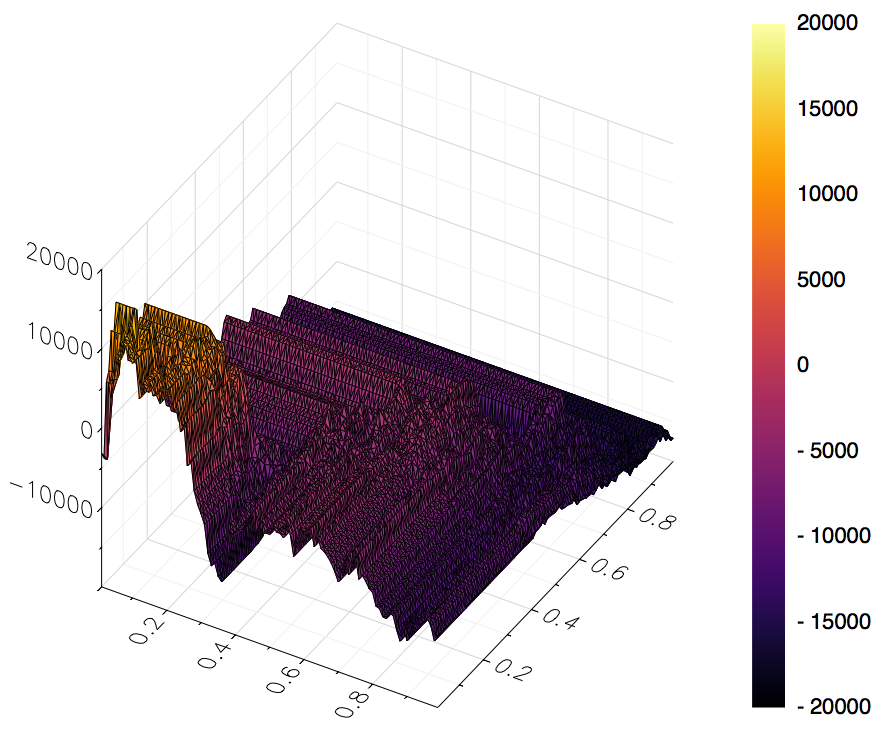66

9

23

3

# Strategems

Strategems is a Julia package aimed at simplifying and streamlining the process of developing, testing, and optimizing algorithmic/systematic trading strategies. This package is inspired in large part by the quantstrat1,2 package in R, adopting a similar general structure to the building blocks that make up a strategy.

Given the highly iterative nature of event-driven trading strategy development, Julia's high-performance design (particularly in the context of loops) and straightforward syntax would seem to make it a natural fit as a language for systematic strategy research and development. While this package remains early in development, with time the hope is to be able to rapidly implement a trading idea, construct a historical backtest, analyze its results, optimize over a given parameter set, and visualize all of this with great detail.

## Dependencies

This package makes heavy use of the Temporal package's `TS` time series type to facilitate the underlying computations involved in cleaning & preprocessing the data used when testing a `Strategy`. Additionally, the Indicators package offers many technical analysis functions that have been written/designed with the goal of a highly generalized systematic trading strategy research engine in mind, and should thus should simplify the process of working with this data quite a bit.

## Install

The Strategems package can be installed using the standard Julia package manager functions.

``````# Option A:

# Option B:
Pkg.clone("https://github.com/dysonance/Strategems.jl")
``````

# Anatomy of a Strategy

Below are the basic building blocks making up the general anatomy of a Strategy with respect to the `Strategems.jl` package design and the type definitions used to facilitate the research workflow.

• `Universe`: encapsulation of the assets/securities the strategy is to be allowed to trade
• `Indicator`: calculation done on each asset in the universe whose results we think have predictive potential for future price movement
• `ParameterSet`: inputs/arguments to the indicator calculations
• `Signal`: boolean flag sending messages to the trading logic/rules to be interpreted and acted upon
• `Rule`: applications of trading logic derived from interpretations of prior calculations & signals at each time step
• `Strategy`: overarching object encapsulating and directing all of the above logic and data to power the backtesting engine

# Example Usage

Below is a quick example demonstrating a simple use-case that one might use to get acquainted with how the package works. Note that the custom infix operators denoted by the uparrow and downarrow below are defined in this package as another way of expressing that one variable crosses over another. The intention of this infix operator definition is to hopefully make the definition of a strategy more syntactically expressive and intuitive.

The key indicator used in this strategy is John Ehlers's MESA Adaptive Moving Average (or MAMA for short). This functionality is implemented in the `Indicators.jl` package described above, and outputs a `Matrix` (or `TS` object if one is passed as an input) of two columns, the first being the MAMA itself and the second being the FAMA, or following adaptive moving average.

This strategy simply goes long when the MAMA crosses over the FAMA, and goes short when the FAMA crosses over the MAMA. Below is an implementation that shows how to set default arguments to the `Indicators.mama` function and run a simple backtest using those parameters, and also define specified ranges over which we might like to see how the strategy behaves under different parameter sets.

``````using Strategems, Indicators, Temporal, Dates

# define universe and gather data
assets = ["CHRIS/CME_CL1", "CHRIS/CME_RB1"]
universe = Universe(assets)
savedata_path = joinpath(dirname(pathof(Strategems)), "..", "data", "\$asset.csv")
if isfile(savedata_path)
else
X = quandl(asset)
if !isdir(dirname(savedata_path))
mkdir(dirname(savedata_path))
end
Temporal.tswrite(X, savedata_path)
end
return X
end
end
gather!(universe, source=datasource)

# define indicators and parameter space
arg_names     = [:fastlimit, :slowlimit]
arg_defaults  = [0.5, 0.05]
arg_ranges    = [0.01:0.01:0.99, 0.01:0.01:0.99]
paramset      = ParameterSet(arg_names, arg_defaults, arg_ranges)
f(x; args...) = Indicators.mama(x; args...)
indicator     = Indicator(f, paramset)

# define signals that will trigger trading decisions
# note the uparrow infix operator is defined to simplify one variable crossing over another
# (similarly for the downarrow infix operator for crossing under)
siglong  = @signal MAMA ↑ FAMA
sigshort = @signal MAMA ↓ FAMA
sigexit  = @signal MAMA == FAMA

longrule  = @rule siglong → long 100
shortrule = @rule sigshort → short 100
exitrule  = @rule sigexit → liquidate 1.0
rules     = (longrule, shortrule, exitrule)

# run strategy
strat = Strategy(universe, indicator, rules)
backtest!(strat)
optimize!(strat, samples=0)  # randomly sample the parameter space (0 -> use all combinations)

# cumulative pnl for each combination of the parameter space
strat.backtest.optimization

# visualizing results with the Plots.jl package
using Plots
gr()
(x, y, z) = (strat.backtest.optimization[:,i] for i in 1:3)
surface(x, y, z)
``````• Get a sufficiently full-featured type system established to facilitate easy construction of simple strategies
• Allow more intelligent logic for trading rules
• Adjust order sizing based on portfolio/account at time t
• Portfolio optimization logic
• Risk limits
• Stop loss rules
• Define a more diverse set of order types
• Limit orders
• Stop orders

09/27/2016

17 days ago

189 commits